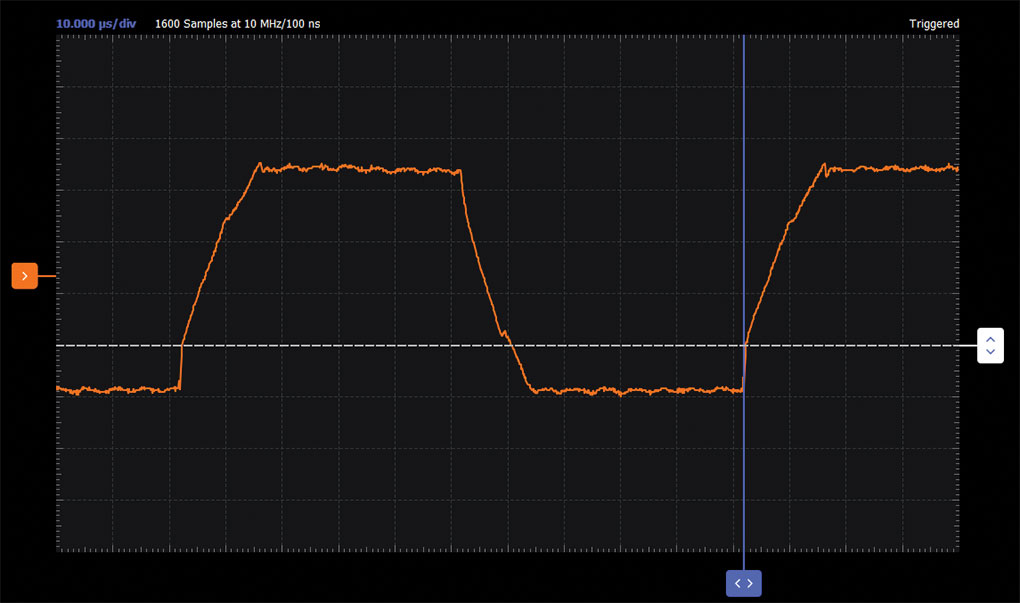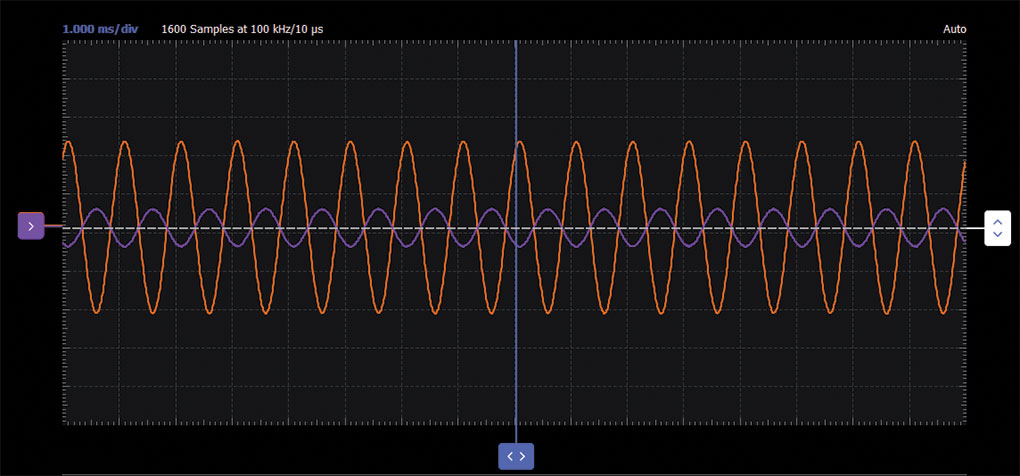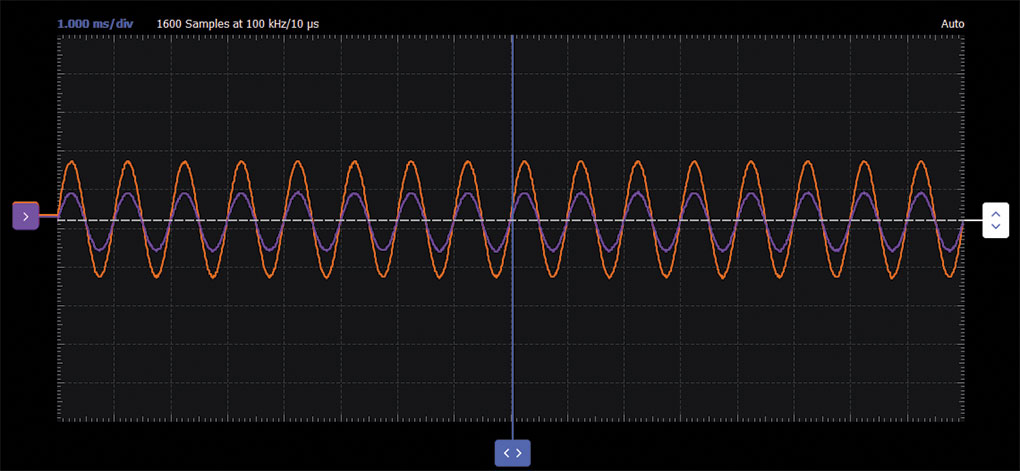# ADALM2000 Simple Op Amps

### Objective:

In this lab we introduce the operational amplifier (op amp), an active circuit that is designed with certain characteristics (high input resistance, low output resistance, and a large differential gain) that make it a nearly ideal amplifier and useful building block in many circuit applications. In this lab you will learn about dc biasing for active circuits and explore a few of the basic functional op amp circuits. We will also use this lab to continue developing skills with the lab hardware.

### Materials:

• ADALM2000 active learning module
• Solderless breadboard and jumper wire kit
• One 1 kΩ resistor
• Two 4.7 kΩ resistors
• Two 10 kΩ resistors
• Two OP97s (low slew rate amplifiers supplied with the recent versions of the ADALP2000 analog parts kit)
• Two 0.1 μF capacitors (radial lead)

### 1.1 Op Amp Basics

#### First Step: Connecting DC Power

Op amps must always be supplied with dc power, therefore it is best to configure these connections first before adding any other circuit components. Figure 1 shows one possible power arrangement on your solderless breadboard. We use two of the long rails for the positive and negative supply voltages, and two others for any ground connections that may be required. Included are the so-called supply decoupling capacitors connected between the power supply and ground rails. It is too early to discuss in great detail the purpose of these capacitors, but they are used to reduce noise on the supply lines and avoid parasitic oscillations. It is considered good practice in analog circuit design to always include small bypass capacitors close to the supply pins of each op amp in your circuit.

Insert the op amp into your breadboard and add the wires and supply capacitors, as shown in Figure 1. To avoid problems later, you may want to attach a small label to the breadboard to indicate which rails correspond to +Vp, –Vn, and ground. Color coding your wires red for Vp, black for Vn, and green for ground can also help to keep the connections organized.

Next, attach the supply and GND connections from the ADALM2000 board to the terminals on your breadboard. Use jumper wires to power the rails as shown. Remember, the power supply GND terminal will be our circuit ground reference. Once you have your supply connections, you may want to use a DMM to probe the IC pins directly to ensure that pin 7 is at 5 V and pin 4 is at –5 V. Remember, you must have the Scopy software running and have turned on the power supplies before measuring the voltages with the voltmeter.

#### Unity-Gain Amplifier (Voltage Follower)

Background:

Our first op amp circuit, shown in Figure 2, is a simple one. This is called a unity-gain buffer, or sometimes just a voltage follower, as defined by the transfer function VOUT = VIN. At first glance, the circuit may seem like a useless device, but as we will show later, it finds use because of its high input resistance and low output resistance.

Hardware Setup:

Using your breadboard and the ADALM2000 power supplies, construct the circuit shown in Figure 3. Note that the power connections have not been explicitly shown here; it is assumed that those connections must be made in any real circuit (as you did in the previous step), so it is unnecessary to show them in the schematic from this point on. Use jumper wires to connect the input and output to the waveform generator and oscilloscope leads. Don’t forget to ground the scope negative input leads C1– and C2– (ground connections are not shown in the schematic).

Procedure:

Use the first waveform generator as source VIN to provide a 2 V amplitude, 1 kHz sine wave excitation to the circuit. Configure the scope so that the input signal is displayed on Channel 2 and the output signal is displayed on Channel 1. Export a plot of the two resulting waveforms and include it in your lab report, noting the parameters of the waveforms (peak values and the fundamental time period or frequency). Your waveforms should confirm the description of this as a unity-gain or voltage follower circuit.

A plot example is presented in Figure 4.Figure 4. Unity-gain follower waveforms.

#### Slew Rate Limitations

For an ideal op amp, the output will follow the input signal precisely for any input signals, but in a real amplifier the output signal can never respond instantaneously to the input signal. This nonideality can be observed when the input signal is a rapidly changing function of time. For large amplitude signals, this limitation is quantified by the slew rate, which is the maximum rate-of-change (slope) of the output voltage that the op amp can deliver. The units of slew rate are usually expressed as V/μs.

Set the waveform generator to a square wave signal with a 2 V amplitude and increase the frequency until you see a significant departure from ideal behavior—that is, when the output starts looking more like a trapezoid than a square wave. You will likely need to adjust the time scale (sec/div) on the scope display to see this. Export a plot of the output waveforms at this point and measure its 10% to 90% rise time (and 90% to 10% fall time) as defined in Figure 5. Also note the peak-to-peak voltage of the output signal. Compute and record the slew rate for both rising and falling outputs according to your measurements. Comment on why the response to rising and falling edges might be different.

A waveform that exemplifies the slew rate is presented in Figure 6.Figure 6. Slew rate waveform.

#### Buffering Example

The high input resistance of the op amp (zero input current) means there is very little loading on the generator; that is, no current is drawn from the source circuit and therefore no voltage drops on any internal Thevenin resistance. Thus, in this configuration, the op amp acts like a buffer to shield the source from the loading effects from other parts of the system. From the perspective of the load circuit, the buffer transforms a nonideal voltage source into a nearly ideal source. Figure 7 describes a simple circuit that we can use to demonstrate this feature of a unity-gain buffer. In the figure, the buffer is inserted between a voltage divider circuit and some load resistance.

Turn off the power supplies and add the resistors to your circuit as shown in Figure 7 (note that we have not changed the op amp connections here, we’ve just flipped the op amp symbol relative to Figure 2).

Turn on the power supplies and set the waveform generator to a 1 kHz sine signal with a 4 V amplitude. Use the scope to simultaneously observe VIN and VOUT and record the amplitudes in your lab report.

Remove the 10 kΩ load and substitute a 1 kΩ resistor instead. Record the amplitude.

Now move the 1 kΩ load between pin 3 and ground so that it is in parallel with the 4.7 kΩ resistor. Record how the output amplitude has changed. Can you predict the new output amplitude?

### 1.2 Simple Amplifier Configurations

#### Inverting Amplifier

Background:

Figure 8 shows the conventional inverting amplifier configuration with a 10 kΩ load resistor at the output.Figure 8. Inverting amplifier configuration.

Hardware Setup:

Now assemble the inverting amplifier circuit shown in Figure 9, where R2 = 4.7 kΩ. Remember to shut off the power supply before assembling a new circuit. Cut and bend the resistor leads as needed to keep them flat against the board surface and use the shortest jumper wires for each connection (as in Figure 1). Remember, the breadboard gives you a lot of flexibility. For example, the leads of resistor R2 do not necessarily have to bridge over the op amp from pin 2 to pin 6; you could use an intermediate node and a jumper wire to go around the device instead.

Turn on the power supplies and observe the current draw to be sure there are no accidental shorts. Now adjust the waveform generator to produce a 2 V amplitude and a 1 kHz sine wave at the input (VIN), and again display both the input and output on the oscilloscope. Measure and record the voltage gain of this circuit and compare it to the theory that was discussed. Export a plot of the input/output waveforms to be included your lab report.

This is a good point to comment on circuit debugging. At some point in the exercise, you are likely to have trouble getting your circuit to work. That is not unexpected, nobody is perfect. However, you should not assume that a nonworking circuit must imply a malfunctioning part or lab instrument. That is almost never true; 99% of all circuit problems are simple wiring or power supply errors. Even experienced engineers will make mistakes from time to time and, consequently, learning how to debug circuit problems is a very important part of the learning process. It is not the TA’s responsibility to diagnose errors for you, and if you find yourself relying on others in this way, then you are missing a key point of the lab and you will be unlikely to succeed in later coursework. Unless smoke is issuing from your op amp or there are brown burn marks on your resistors or your capacitor has exploded, your components are probably fine. In fact, most of them can tolerate a little abuse before significant damage is done. The best thing to do when things aren’t working is to turn off the power supplies and look for a simple explanation before blaming parts or equipment. The DMM can be a valuable debugging tool in this regard.

Procedure:

Use the first waveform generator as source VIN to provide a 2 V amplitude, 1 kHz sine wave excitation to the circuit. Configure the scope so that the input signal is displayed on Channel 2 and the output signal is displayed on Channel 1.

A plot example is presented in Figure 10.Figure 10. Inverting amplifier waveforms.

#### Output Saturation

Now change the feedback resistor R2 in Figure 8 from 4.7 kΩ to 10 kΩ. What is the gain now? Slowly increase the amplitude of the input signal to 2 V and export the waveforms into your lab notebook. The output voltage of any op amp is ultimately limited by the supply voltages, and in many cases the actual limits are much smaller than the supply voltages due to internal voltage drops in the circuitry. Quantify the internal voltage drops in the OP97 based on your measurements.

#### Summing Amplifier Circuit

Background:

The circuit of Figure 11 is a basic inverting amplifier with an additional input, called a summing amplifier. Using superposition we can show that VOUT is a linear sum of VIN1 and VIN2, each with their own unique gain or scale factor.Figure 11. Summing amplifier configuration.

Hardware Setup:

With the power turned off, modify your inverting amplifier circuit as shown in Figure 12. Use the second waveform generator output for VIN2. Turn the amplitude all the way to zero so that you can adjust up from zero during the experiment.

Now apply a 2 V amplitude sine wave for VIN1 and 1 V dc for VIN2. Observe and record the input/output waveforms on the oscilloscope screen. Pay close attention to the ground signal level of the output channel on the oscilloscope screen. When used in this way, such a circuit can be called a level shifter.

Adjust the dc offset of waveform generator W1 (VIN1) until VOUT has zero dc component. Estimate the required dc offset by observing the input waveform on the scope (note: it is not –VIN2).

Reset the offset of waveform generator W1 to zero. With Channel 2 of the scope (the channel connected to the op amp output), set for 2 V/div and slowly increase the offset voltage of waveform generator W2, VIN2. What happens to VOUT? Record the dc voltage of the output.

Return the offset voltage of waveform generator W2 to approximately 1 V. Set the scope to 1 V/div and adjust the scope offset so you can see the complete VOUT waveform. Turn VIN2 back up to the value you increased it to in the previous step. What does the oscilloscope trace for VOUT look like? Does the amplifier appear to be amplifying?

Procedure:

Use the first waveform generator as source VIN to provide a 2 V amplitude, 1 kHz sine wave excitation to the circuit. The second waveform generator is used to generate 1 V constant voltage. Configure the scope so that the input signal is displayed on Channel 2 and the output signal is displayed on Channel 1.

A plot example is presented in Figure 13.Figure 13. Summing amplifier waveforms.

#### Noninverting Amplifier

Background:

The noninverting amplifier configuration is shown in Figure 14. Like the unity-gain buffer, this circuit has the (usually) desirable property of high input resistance, so it is useful for buffering nonideal sources:Figure 14. Noninverting amplifier with gain.

Hardware Setup:

Assemble the noninverting amplifier circuit shown in Figure 15. Remember to shut off the power supplies before assembling the new circuit. Start with R2 = 1 kΩ.

Apply a 2 V amplitude, 1 kHz sine wave at the input, and display both input and output on the scope. Measure the voltage gain of this circuit and compare it to the theory discussed before. Export a plot of the waveforms and include it in your lab report.

Increase the feedback resistor (R2) from 1 kΩ to about 5 kΩ. What is the gain now?

Increase the feedback resistance further until the onset of clipping, that is, until the peaks of the output signal begin to be flattened due to output saturation. Record the value of resistance where this happens. Now increase the feedback resistance to 100 kΩ. Describe and draw waveforms in your notebook. What is the theoretical gain at this point? How small would the input signal have to be in order to keep the output level to less than 5 V given this gain? Try to adjust the waveform generator to this value. Describe the output achieved.

The last step underscores an important consideration for high gain amplifiers. High gain necessarily implies a large output for a small input level. Sometimes this can lead to inadvertent saturation due to the amplification of some low level noise or interference—for example, the amplification of stray 60 Hz signals from power lines that can sometimes be picked up. Amplifiers will amplify any signals at the input terminals, whether you want them to or not!

Procedure:

Use the first waveform generator as source VIN to provide a 2 V amplitude, 1 kHz sine wave excitation to the circuit. Configure the scope so that the input signal is displayed on Channel 2 and the output signal is displayed on Channel 1.

A plot example is presented in Figure 16.Figure 16. Noninverting amplifier waveforms.

### Questions

• Considering the inverting amplifier in Figure 8, compute the gain as a function dependent on R1 = 1 kΩ and R2 = 4.7 kΩ.
• Considering the noninverting amplifier in Figure 14, compute the gain as a function dependent on R1 = 1 kΩ and R2 = 1 kΩ.

You can find the answers at the StudentZone blog.